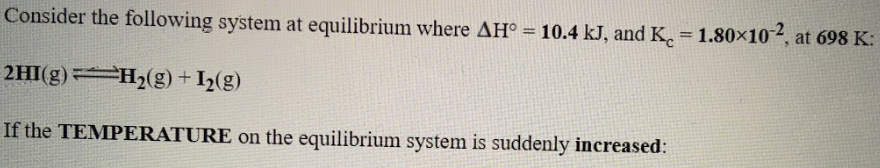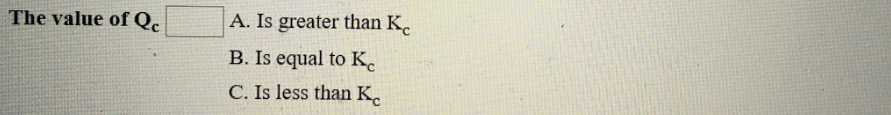# Consider the following system at equilibrium where ΔH° = 10.4 kJ, and Kc = 1.80x10^-2, at 698 K. 2HI(g) ⇌ H2(g) + I2(g) If the TEMPERATURE on the equilibrium system is suddenly increased: The value of Qc a) Is greater than Kc b) Is equal to Kc c) is less than Kc